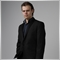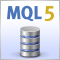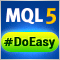# 继续漫步优化（第二部分）：为任意机器人创建优化报告的机制

24 二月 2020, 11:140
1 169

### 自定义佣金和滑点的实现

MetaTrader 5 平台测试器提供了许多令人兴奋的可能性。 然而，某些经纪商未将交易佣金添加到历史记录中。 进而，有时您也许要附加佣金以便进行额外的策略测试。 为此目的，我已新加了一个类，可为每个单独的品种保存佣金。 调用相应的方法后，该类将返回佣金和指定的滑点。 该类本身清单如下：

```class CCCM
{
private:
struct Keeper
{
string            symbol;
double            comission;
double            shift;
};

Keeper            comission_data[];
public:

double            get(string symbol,double price,double volume);
void              remove(string symbol);
};

```

```void CCCM::add(string symbol,double comission,double shift)
{
int s=ArraySize(comission_data);

for(int i=0;i<s;i++)
{
if(comission_data[i].symbol==symbol)
return;
}

ArrayResize(comission_data,s+1,s+1);

Keeper keeper;
keeper.symbol=symbol;
keeper.comission=MathAbs(comission);
keeper.shift=MathAbs(shift);

comission_data[s]=keeper;
}

```

• 佣金：根据资产类型，佣金可以按获利货币、或交易量的百分比加收。
• 滑点：始终按点数为单位指定。

remove 方法删除所选资产。 品种名作为关键字。

```void CCCM::remove(string symbol)
{
int total=ArraySize(comission_data);
int ind=-1;
for(int i=0;i<total;i++)
{
if(comission_data[i].symbol==symbol)
{
ind=i;
break;
}
}
if(ind!=-1)
ArrayRemove(comission_data,ind,1);
}

```

get 方法用于获取所选的偏移值和佣金。 针对不同的资产类型，该方法的实现方式是不同的。

```double CCCM::get(string symbol,double price,double volume)
{

int total=ArraySize(comission_data);
for(int i=0;i<total;i++)
{
if(comission_data[i].symbol==symbol)
{

double ans;
switch(mode)
{
case SYMBOL_CALC_MODE_FOREX :
ans=(comission_data[i].comission+shift)*volume;
break;
case SYMBOL_CALC_MODE_FOREX_NO_LEVERAGE :
ans=(comission_data[i].comission+shift)*volume;
break;
case SYMBOL_CALC_MODE_FUTURES :
ans=(comission_data[i].comission+shift)*volume;
break;
case SYMBOL_CALC_MODE_CFD :
ans=(comission_data[i].comission+shift)*volume;
break;
case SYMBOL_CALC_MODE_CFDINDEX :
ans=(comission_data[i].comission+shift)*volume;
break;
case SYMBOL_CALC_MODE_CFDLEVERAGE :
ans=(comission_data[i].comission+shift)*volume;
break;
case SYMBOL_CALC_MODE_EXCH_STOCKS :
{
}
break;
case SYMBOL_CALC_MODE_EXCH_FUTURES :
ans=(comission_data[i].comission+shift)*volume;
break;
case SYMBOL_CALC_MODE_EXCH_FUTURES_FORTS :
ans=(comission_data[i].comission+shift)*volume;
break;
case SYMBOL_CALC_MODE_EXCH_BONDS :
{
}
break;
case SYMBOL_CALC_MODE_EXCH_STOCKS_MOEX :
{
}
break;
case SYMBOL_CALC_MODE_EXCH_BONDS_MOEX :
{
}
break;
case SYMBOL_CALC_MODE_SERV_COLLATERAL :
ans=(comission_data[i].comission+shift)*volume;
break;
default: ans=0; break;
}

if(ans!=0)
return -ans;

}
}

return 0;
}

```

### 在 CDealHistoryGetter 类里的创新

• getHistory — 此方法允许下载按仓位分组的交易历史记录。 如果我们在循环里用标准方法下载交易历史记录，且不用任何过滤器，则我们会收到按照 DealData 结构呈现的交易描述：

```struct DealData
{
long              ticket;        // Deal ticket
long              order;         // The number of the order that opened the position
datetime          DT;            // Position open date
long              DT_msc;        // Position open date in milliseconds
ENUM_DEAL_TYPE    type;          // Open position type
ENUM_DEAL_ENTRY   entry;         // Position entry type
long              magic;         // Unique position number
ENUM_DEAL_REASON  reason;        // Order placing reason
long              ID;            // Position ID
double            volume;        // Position volume (lots)
double            price;         // Position entry price
double            comission;     // Commission paid
double            swap;          // Swap
double            profit;        // Profit / loss
string            symbol;        // Symbol
string            comment;       // Comment specified when at opening
string            ID_external;   // External ID
};

```

```struct DealKeeper
{
DealData          deals[]; /* List of all deals for this position
(or several positions in case of position reversal)*/
string            symbol;  // Symbol
long              ID;      // ID of the position (s)
datetime          DT_min;  // Open date (or the date of the very first position)
datetime          DT_max;  // Close date
};

```

```#ifndef ONLY_CUSTOM_COMISSION
if(data.comission==0 && comission_manager != NULL)
{
data.comission=comission_manager.get(data.symbol,data.price,data.volume);
}
#else
data.comission=comission_manager.get(data.symbol,data.price,data.volume);
#endif

```

• getIDArr — 返回在请求的时间范围内为所有交易品种的开仓 ID 数组。 仓位 ID 可以将所有成交合并到我们方法中的仓位。 实际上，这是 DealData.ID 字段的唯一列表。
• getDealsDetales — 该方法类似于 getHistory，但是它提供的细节较少。 该方法的思路是提供一种易于阅读的仓位列表，其中每一行对应一笔特定的成交。 每笔仓位由以下结构描述：
```struct DealDetales
{
string            symbol;        // Symbol
datetime          DT_open;       // Open date
ENUM_DAY_OF_WEEK  day_open;      // Open day
datetime          DT_close;      // Cloe date
ENUM_DAY_OF_WEEK  day_close;     // Close day
double            volume;        // Volume (lots)
bool              isLong;        // Long/Short
double            price_in;      // Position entry price
double            price_out;     // Position exit price
double            pl_oneLot;     // Profit / loss is trading one lot
double            pl_forDeal;    // Real profit/loss taking into account commission
string            open_comment;  // Comment at the time of opening
string            close_comment; // Comment at the time of closing
};

```
它们代表按平仓日期排序的仓位表。 这些数值的数组将在接下来的类中用于计算系数。 另外，我们还将得到基于所示数据得最终测试报告。 甚而，基于此类数据，测试器可以在交易后创建盈亏曲线图。

对于测试器，请注意，在进一步的计算中，终端计算出的恢复因子会与根据接收数据计算出的恢复因子有所不同。 这一事实出于尽管数据下载正确，且计算公式相同，但源数据却不同。 测试器使用绿线（即详细报告）计算恢复因子，而我们将使用蓝线（即忽略开仓和平仓之间发生的价格波动数据）进行计算。
• getBalance — 此方法旨在获取余额数据，且不考虑指定日期的交易操作。
```double CDealHistoryGetter::getBalance(datetime toDate)
{
if(HistorySelect(0,(toDate>0 ? toDate : TimeCurrent())))
{
int total=HistoryDealsTotal(); // Get the total number of positions
double balance=0;
for(int i=0; i<total; i++)
{
long ticket=(long)HistoryDealGetTicket(i);

ENUM_DEAL_TYPE dealType=(ENUM_DEAL_TYPE)HistoryDealGetInteger(ticket,DEAL_TYPE);
if(dealType==DEAL_TYPE_BALANCE ||
dealType == DEAL_TYPE_CORRECTION ||
dealType == DEAL_TYPE_COMMISSION)
{
balance+=HistoryDealGetDouble(ticket,DEAL_PROFIT);

if(toDate<=0)
break;
}
}
return balance;
}
else
return 0;
}

```

• getBalanceWithPL — 该方法与前一种方法类似，但它另行考虑了所执行操作的盈亏造成的对余额变化，包括根据上述原则的佣金。

### 创建优化报告的类 — 计算中使用的结构

PL_Keeper 结构:

```struct PL_keeper
{
PLChart_item      PL_total[];
PLChart_item      PL_oneLot[];
PLChart_item      PL_Indicative[];
};

```

`PL_keeper         PL,PL_hist,BH,BH_hist;`

DailyPL_keeper structure:

```// The structure of Daily PL graphs
struct DailyPL_keeper
{
DailyPL           avarage_open,avarage_close,absolute_open,absolute_close;
};

```

```// Table structure of extreme points
struct RatioTable_keeper
{
ProfitDrawdown    Total_max,Total_absolute,Total_percent;
ProfitDrawdown    OneLot_max,OneLot_absolute,OneLot_percent;
};

```

```struct ProfitDrawdown
{
double            Profit; // In some cases Profit, in other Profit / Loss
double            Drawdown; // Drawdown
};

```

```// Structures for calculating consecutive profits and losses
struct S_dealsCounter
{
int               Profit,DD;
};
struct S_dealsInARow : public S_dealsCounter
{
S_dealsCounter    Counter;
};
// Structures for calculating auxiliary data
struct CalculationData_item
{
S_dealsInARow     dealsCounter;
int               R_arr[];
double            DD_percent;
double            Accomulated_DD,Accomulated_Profit;
double            PL;
double            Max_DD_forDeal,Max_Profit_forDeal;
double            Max_DD_byPL,Max_Profit_byPL;
datetime          DT_Max_DD_byPL,DT_Max_Profit_byPL;
datetime          DT_Max_DD_forDeal,DT_Max_Profit_forDeal;
int               Total_DD_numDeals,Total_Profit_numDeals;
};
struct CalculationData
{
CalculationData_item total,oneLot;
int               num_deals;
bool              isNot_firstDeal;
};

```

S_dealsCounter 和 S_dealsInARowow 结构本质上是单个实例。 这种关联和继承的奇怪组合与参数的特定计算有关。 创建 S_dealsInARowow 结构用于存储并计算连串数量的交易（实际上是为了计算仓位，即从开仓到平仓），既有盈利也有亏损。 声明的 S_dealsCounter 结构嵌套实例，用于存储中间计算结果。 继承的字段存储总和。 稍后我们将返回到计算盈利/亏损成交的操作。

CalculationData_item 结构包含计算系数所需的字段。

• R_arr — 连续盈利/亏损成交序列的数组，分别显示为 1 / 0。 该数组用于 Z 分数计算。
• DD_percent — 回撤百分比。
• Accomulated_DD, Accomulated_Profit  — 存储亏损和利润值总和。
• PL — 盈利 / 亏损。
• Max_DD_forDeal, Max_Profit_forDeal — 顾名思义，它们存储所有成交中的最大亏损和盈利。
• Max_DD_byPL, Mаx_Profit_byPL — 存储由盈亏图计算出的最大亏损和利润。
• DT_Max_DD_byPL, DT_Max_Profit_byPL — 存储由盈亏图得来的最高亏损和最高盈利的日期。
• DT_Max_DD_forDeal, DT_Max_Profit_forDeal — 最高亏损和获利成交的日期。
• Total_DD_numDeals, TotalProfit_numDeals — 获利和亏损交易的总数。

CalculationData 是一个累加结构，它合并了所有描述过的结构。 它存储所有必需的数据。 它还包含 num_deals 字段，该字段实际上是 CalculationData_item::Total_DD_numDeals 和 CalculationData_item::TotalProfit_numDeals 的总和。 sNot_firstDeal 字段是一个技术标志，它意味着本次计算并非是第一笔成交。

CoefChart_keeper 结构:

```struct CoefChart_keeper
{
CoefChart_item    OneLot_ShartRatio_chart[],Total_ShartRatio_chart[];
CoefChart_item    OneLot_WinCoef_chart[],Total_WinCoef_chart[];
CoefChart_item    OneLot_RecoveryFactor_chart[],Total_RecoveryFactor_chart[];
CoefChart_item    OneLot_ProfitFactor_chart[],Total_ProfitFactor_chart[];
CoefChart_item    OneLot_AltmanZScore_chart[],Total_AltmanZScore_chart[];
};

```

СHistoryComparer 类：

```class CHistoryComparer : public ICustomComparer<DealDetales>
{
public:
int               Compare(DealDetales &x,DealDetales &y);
};

```

```int CReportCreator::CHistoryComparer::Compare(DealDetales &x,DealDetales &y)
{
return(x.DT_close == y.DT_close ? 0 : (x.DT_close > y.DT_close ? 1 : -1));
}

```

```PL_detales        PL_detales_data;
DistributionChart OneLot_PDF_chart,Total_PDF_chart;

```

PL_detales 结构包含有关获利和亏损仓位的简要交易信息：

```//+------------------------------------------------------------------+
struct PL_detales_PLDD
{
int               orders; // Number of deals
double            orders_in_Percent; // Number of orders as % of total number of orders
int               dealsInARow; // Deals in a row
double            totalResult; // Total result in money
double            averageResult; // Average result in money
};
//+------------------------------------------------------------------+
//|                                                                  |
//+------------------------------------------------------------------+
struct PL_detales_item
{
PL_detales_PLDD   profit; // Information on profitable deals
PL_detales_PLDD   drawdown; // Information on losing deals
};
//+-------------------------------------------------------------------+
//| A brief PL graph summary divided into 2 main blocks               |
//+-------------------------------------------------------------------+
struct PL_detales
{
PL_detales_item   total,oneLot;
};

```

```//+------------------------------------------------------------------+
//| Structure used for saving distribution charts                    |
//+------------------------------------------------------------------+
struct Chart_item
{
double            y; // y axis
double            x; // x axis
};
//+------------------------------------------------------------------+
//| Structure contains the VaR value                                 |
//+------------------------------------------------------------------+
struct VAR
{
double            VAR_90,VAR_95,VAR_99;
double            Mx,Std;
};
//+------------------------------------------------------------------+
//| Structure - it is used to store distribution charts and          |
//| the VaR values                                                   |
//+------------------------------------------------------------------+
struct Distribution_item
{
Chart_item        distribution[]; // Distribution chart
VAR               VaR; // VaR
};
//+------------------------------------------------------------------+
//| Structure - Stores distribution data. Divided into 2 blocks      |
//+------------------------------------------------------------------+
struct DistributionChart
{
Distribution_item absolute,growth;
};

```

VaR 系数是根据以下公式计算得出：历史 VaR，可能不够准确，但是非常适合当前的实现。

### 计算系数的方法可以描述交易结果

```void CReportCreator::CalcPL(const DealDetales &deal,CalculationData &data,PLChart_item &pl_out[],CalcType type)
{
PLChart_item item;
ZeroMemory(item);
item.DT=deal.DT_close; // Saving the date

if(type!=_Indicative)
{
item.Profit=(type==_Total ? data.total.PL : data.oneLot.PL); // Saving the profit
item.Drawdown=(type==_Total ? data.total.DD_percent : data.oneLot.DD_percent); // Saving the drawdown
}
else // Calculating the indicative chart
{
if(data.isNot_firstDeal)
{
if(data.total.PL!=0)
{
if(data.total.PL > 0 && data.total.Max_DD_forDeal < 0)
item.Profit=data.total.PL/MathAbs(data.total.Max_DD_forDeal);
else
if(data.total.PL<0 && data.total.Max_Profit_forDeal>0)
item.Profit=data.total.PL/data.total.Max_Profit_forDeal;
}
}
}
int s=ArraySize(pl_out);
ArrayResize(pl_out,s+1,s+1);
pl_out[s]=item;
}

```

• 如果盈亏大于零且回撤较小，则将当前盈亏值除以回撤值。 因此，我们获得一个系数，该系数表示为了将当前盈亏减至零，需要多少连续的最大回撤次数。
• 如果盈亏小于零，且所有成交的最大利润都大于零，则我们将盈亏值（当前为亏损）除以获得的最大利润。 由此，我们得到一个系数，该系数表示为了将当前回撤降至零，需要多少连续的最大盈利次数。

CalcData 和 CalcData_item:

```//+------------------------------------------------------------------+
//| Calculating auxiliary data                                       |
//+------------------------------------------------------------------+
void CReportCreator::CalcData_item(const DealDetales &deal,CalculationData_item &out,
bool isOneLot)
{
double pl=(isOneLot ? deal.pl_oneLot : deal.pl_forDeal); //PL
int n=0;
// Number of profits and losses
if(pl>=0)
{
out.Total_Profit_numDeals++;
n=1;
out.dealsCounter.Counter.DD=0;
out.dealsCounter.Counter.Profit++;
}
else
{
out.Total_DD_numDeals++;
out.dealsCounter.Counter.DD++;
out.dealsCounter.Counter.Profit=0;
}
out.dealsCounter.DD=MathMax(out.dealsCounter.DD,out.dealsCounter.Counter.DD);
out.dealsCounter.Profit=MathMax(out.dealsCounter.Profit,out.dealsCounter.Counter.Profit);

// Series of profits and losses
int s=ArraySize(out.R_arr);
if(!(s>0 && out.R_arr[s-1]==n))
{
ArrayResize(out.R_arr,s+1,s+1);
out.R_arr[s]=n;
}

out.PL+=pl; //Total PL
// Max Profit / DD
if(out.Max_DD_forDeal>pl)
{
out.Max_DD_forDeal=pl;
out.DT_Max_DD_forDeal=deal.DT_close;
}
if(out.Max_Profit_forDeal<pl)
{
out.Max_Profit_forDeal=pl;
out.DT_Max_Profit_forDeal=deal.DT_close;
}
// Accumulated Profit / DD
out.Accomulated_DD+=(pl>0 ? 0 : pl);
out.Accomulated_Profit+=(pl>0 ? pl : 0);
// Extreme profit values
double maxPL=MathMax(out.Max_Profit_byPL,out.PL);
if(compareDouble(maxPL,out.Max_Profit_byPL)==1/* || !isNot_firstDeal*/)// another check is needed to save the date
{
out.DT_Max_Profit_byPL=deal.DT_close;
out.Max_Profit_byPL=maxPL;
}
double maxDD=out.Max_DD_byPL;
double DD=0;
if(out.PL>0)
DD=out.PL-maxPL;
else
DD=-(MathAbs(out.PL)+maxPL);
maxDD=MathMin(maxDD,DD);
if(compareDouble(maxDD,out.Max_DD_byPL)==-1/* || !isNot_firstDeal*/)// another check is needed to save the date
{
out.Max_DD_byPL=maxDD;
out.DT_Max_DD_byPL=deal.DT_close;
}
out.DD_percent=(balance>0 ?(MathAbs(DD)/(maxPL>0 ? maxPL : balance)) :(maxPL>0 ?(MathAbs(DD)/maxPL) : 0));
}

```

CalcData 数据用得到的中间数据来计算所需的系数，并在每次迭代时更新计算。 它的实现如下：

```void CReportCreator::CalcData(const DealDetales &deal,CalculationData &out,bool isBH)
{
out.num_deals++; // Counting the number of deals
CalcData_item(deal,out.oneLot,true);
CalcData_item(deal,out.total,false);

if(!isBH)
{
// Fill PL graphs
CalcPL(deal,out,PL.PL_total,_Total);
CalcPL(deal,out,PL.PL_oneLot,_OneLot);
CalcPL(deal,out,PL.PL_Indicative,_Indicative);

// Fill PL Histogram graphs
CalcPLHist(deal,out,PL_hist.PL_total,_Total);
CalcPLHist(deal,out,PL_hist.PL_oneLot,_OneLot);
CalcPLHist(deal,out,PL_hist.PL_Indicative,_Indicative);

// Fill PL graphs by days
CalcDailyPL(DailyPL_data.absolute_close,CALC_FOR_CLOSE,deal);
CalcDailyPL(DailyPL_data.absolute_open,CALC_FOR_OPEN,deal);
CalcDailyPL(DailyPL_data.avarage_close,CALC_FOR_CLOSE,deal);
CalcDailyPL(DailyPL_data.avarage_open,CALC_FOR_OPEN,deal);

// Fill Profit Factor graphs
ProfitFactor_chart_calc(CoefChart_data.OneLot_ProfitFactor_chart,out,deal,true);
ProfitFactor_chart_calc(CoefChart_data.Total_ProfitFactor_chart,out,deal,false);

// Fill Recovery Factor graphs
RecoveryFactor_chart_calc(CoefChart_data.OneLot_RecoveryFactor_chart,out,deal,true);
RecoveryFactor_chart_calc(CoefChart_data.Total_RecoveryFactor_chart,out,deal,false);

// Fill winning coefficient graphs
WinCoef_chart_calc(CoefChart_data.OneLot_WinCoef_chart,out,deal,true);
WinCoef_chart_calc(CoefChart_data.Total_WinCoef_chart,out,deal,false);

// Fill Sharpe Ration graphs
ShartRatio_chart_calc(CoefChart_data.OneLot_ShartRatio_chart,PL.PL_oneLot,deal/*,out.isNot_firstDeal*/);
ShartRatio_chart_calc(CoefChart_data.Total_ShartRatio_chart,PL.PL_total,deal/*,out.isNot_firstDeal*/);

// Fill Z Score graphs
AltmanZScore_chart_calc(CoefChart_data.OneLot_AltmanZScore_chart,(double)out.num_deals,
(double)ArraySize(out.oneLot.R_arr),(double)out.oneLot.Total_Profit_numDeals,
(double)out.oneLot.Total_DD_numDeals/*,out.isNot_firstDeal*/,deal);
AltmanZScore_chart_calc(CoefChart_data.Total_AltmanZScore_chart,(double)out.num_deals,
(double)ArraySize(out.total.R_arr),(double)out.total.Total_Profit_numDeals,
(double)out.total.Total_DD_numDeals/*,out.isNot_firstDeal*/,deal);
}
else // Fill PL Buy and Hold graphs
{
CalcPL(deal,out,BH.PL_total,_Total);
CalcPL(deal,out,BH.PL_oneLot,_OneLot);
CalcPL(deal,out,BH.PL_Indicative,_Indicative);

CalcPLHist(deal,out,BH_hist.PL_total,_Total);
CalcPLHist(deal,out,BH_hist.PL_oneLot,_OneLot);
CalcPLHist(deal,out,BH_hist.PL_Indicative,_Indicative);
}

if(!out.isNot_firstDeal)
out.isNot_firstDeal=true; // Flag "It is NOT the first deal"
}

```

```//+------------------------------------------------------------------+
//| Create a structure of trading during a day                       |
//+------------------------------------------------------------------+
void CReportCreator::CalcDailyPL(DailyPL &out,DailyPL_calcBy calcBy,const DealDetales &deal)
{
cmpDay(deal,MONDAY,out.Mn,calcBy);
cmpDay(deal,TUESDAY,out.Tu,calcBy);
cmpDay(deal,WEDNESDAY,out.We,calcBy);
cmpDay(deal,THURSDAY,out.Th,calcBy);
cmpDay(deal,FRIDAY,out.Fr,calcBy);
}
//+------------------------------------------------------------------+
//| Save resulting PL/DD for the day                                 |
//+------------------------------------------------------------------+
void CReportCreator::cmpDay(const DealDetales &deal,ENUM_DAY_OF_WEEK etalone,PLDrawdown &ans,DailyPL_calcBy calcBy)
{
ENUM_DAY_OF_WEEK day=(calcBy==CALC_FOR_CLOSE ? deal.day_close : deal.day_open);
if(day==etalone)
{
if(deal.pl_forDeal>0)
{
ans.Profit+=deal.pl_forDeal;
}
else
if(deal.pl_forDeal<0)
{
ans.Drawdown+=MathAbs(deal.pl_forDeal);
}
}
}
//+------------------------------------------------------------------+
//| Average resulting PL/DD for the day                              |
//+------------------------------------------------------------------+
void CReportCreator::avarageDay(PLDrawdown &day)
{
}

```

```//+------------------------------------------------------------------+
//| Calculate Profit Factor                                          |
//+------------------------------------------------------------------+
void CReportCreator::ProfitFactor_chart_calc(CoefChart_item &out[],CalculationData &data,const DealDetales &deal,bool isOneLot)
{
CoefChart_item item;
item.DT=deal.DT_close;
double profit=(isOneLot ? data.oneLot.Accomulated_Profit : data.total.Accomulated_Profit);
double dd=MathAbs(isOneLot ? data.oneLot.Accomulated_DD : data.total.Accomulated_DD);
if(dd==0)
item.coef=0;
else
item.coef=profit/dd;
int s=ArraySize(out);
ArrayResize(out,s+1,s+1);
out[s]=item;
}

```

```//+------------------------------------------------------------------+
//| Calculate Recovery Factor                                        |
//+------------------------------------------------------------------+
void CReportCreator::RecoveryFactor_chart_calc(CoefChart_item &out[],CalculationData &data,const DealDetales &deal,bool isOneLot)
{
CoefChart_item item;
item.DT=deal.DT_close;
double pl=(isOneLot ? data.oneLot.PL : data.total.PL);
double dd=MathAbs(isOneLot ? data.oneLot.Max_DD_byPL : data.total.Max_DD_byPL);
if(dd==0)
item.coef=0;//ideally it should be plus infinity
else
item.coef=pl/dd;
int s=ArraySize(out);
ArrayResize(out,s+1,s+1);
out[s]=item;
}

```

```//+------------------------------------------------------------------+
//| Calculate Win Rate                                               |
//+------------------------------------------------------------------+
void CReportCreator::WinCoef_chart_calc(CoefChart_item &out[],CalculationData &data,const DealDetales &deal,bool isOneLot)
{
CoefChart_item item;
item.DT=deal.DT_close;
double profit=(isOneLot ? data.oneLot.Accomulated_Profit : data.total.Accomulated_Profit);
double dd=MathAbs(isOneLot ? data.oneLot.Accomulated_DD : data.total.Accomulated_DD);
int n_profit=(isOneLot ? data.oneLot.Total_Profit_numDeals : data.total.Total_Profit_numDeals);
int n_dd=(isOneLot ? data.oneLot.Total_DD_numDeals : data.total.Total_DD_numDeals);
if(n_dd == 0 || n_profit == 0)
item.coef = 0;
else
item.coef=(profit/n_profit)/(dd/n_dd);
int s=ArraySize(out);
ArrayResize(out,s+1,s+1);
out[s]=item;
}

```

```//+------------------------------------------------------------------+
//| Calculate Sharpe Ratio                                           |
//+------------------------------------------------------------------+
double CReportCreator::ShartRatio_calc(PLChart_item &data[])
{
int total=ArraySize(data);
double ans=0;
if(total>=2)
{
double pl_r=0;
int n=0;
for(int i=1; i<total; i++)
{
if(data[i-1].Profit!=0)
{
pl_r+=(data[i].Profit-data[i-1].Profit)/data[i-1].Profit;
n++;
}
}
if(n>=2)
pl_r/=(double)n;
double std=0;
n=0;
for(int i=1; i<total; i++)
{
if(data[i-1].Profit!=0)
{
std+=MathPow((data[i].Profit-data[i-1].Profit)/data[i-1].Profit-pl_r,2);
n++;
}
}
if(n>=2)
std=MathSqrt(std/(double)(n-1));

ans=(std!=0 ?(pl_r-r)/std : 0);
}
return ans;
}

```

```//+------------------------------------------------------------------+
//| Distribution calculation                                         |
//+------------------------------------------------------------------+
void CReportCreator::NormalPDF_chart_calc(DistributionChart &out,PLChart_item &data[])
{
double Mx_absolute=0,Mx_growth=0,Std_absolute=0,Std_growth=0;
int total=ArraySize(data);
ZeroMemory(out.absolute);
ZeroMemory(out.growth);
ZeroMemory(out.absolute.VaR);
ZeroMemory(out.growth.VaR);
ArrayFree(out.absolute.distribution);
ArrayFree(out.growth.distribution);

// Calculation of distribution parameters
if(total>=2)
{
int n=0;
for(int i=0; i<total; i++)
{
Mx_absolute+=data[i].Profit;
if(i>0 && data[i-1].Profit!=0)
{
Mx_growth+=(data[i].Profit-data[i-1].Profit)/data[i-1].Profit;
n++;
}
}
Mx_absolute/=(double)total;
if(n>=2)
Mx_growth/=(double)n;

n=0;
for(int i=0; i<total; i++)
{
Std_absolute+=MathPow(data[i].Profit-Mx_absolute,2);
if(i>0 && data[i-1].Profit!=0)
{
Std_growth+=MathPow((data[i].Profit-data[i-1].Profit)/data[i-1].Profit-Mx_growth,2);
n++;
}
}
Std_absolute=MathSqrt(Std_absolute/(double)(total-1));
if(n>=2)
Std_growth=MathSqrt(Std_growth/(double)(n-1));

// Calculate VaR
out.absolute.VaR.Mx=Mx_absolute;
out.absolute.VaR.Std=Std_absolute;
out.absolute.VaR.VAR_90=VaR(Q_90,Mx_absolute,Std_absolute);
out.absolute.VaR.VAR_95=VaR(Q_95,Mx_absolute,Std_absolute);
out.absolute.VaR.VAR_99=VaR(Q_99,Mx_absolute,Std_absolute);
out.growth.VaR.Mx=Mx_growth;
out.growth.VaR.Std=Std_growth;
out.growth.VaR.VAR_90=VaR(Q_90,Mx_growth,Std_growth);
out.growth.VaR.VAR_95=VaR(Q_95,Mx_growth,Std_growth);
out.growth.VaR.VAR_99=VaR(Q_99,Mx_growth,Std_growth);

// Calculate distribution
for(int i=0; i<total; i++)
{
Chart_item  item_a,item_g;
ZeroMemory(item_a);
ZeroMemory(item_g);
item_a.x=data[i].Profit;
item_a.y=PDF_calc(Mx_absolute,Std_absolute,data[i].Profit);
if(i>0)
{
item_g.x=(data[i-1].Profit != 0 ?(data[i].Profit-data[i-1].Profit)/data[i-1].Profit : 0);
item_g.y=PDF_calc(Mx_growth,Std_growth,item_g.x);
}
int s=ArraySize(out.absolute.distribution);
ArrayResize(out.absolute.distribution,s+1,s+1);
out.absolute.distribution[s]=item_a;
s=ArraySize(out.growth.distribution);
ArrayResize(out.growth.distribution,s+1,s+1);
out.growth.distribution[s]=item_g;
}
// Ascending
sorter.Sort<Chart_item>(out.absolute.distribution,&chartComparer);
sorter.Sort<Chart_item>(out.growth.distribution,&chartComparer);
}
}
//+------------------------------------------------------------------+
//| Calculate VaR                                                    |
//+------------------------------------------------------------------+
double CReportCreator::VaR(double quantile,double Mx,double Std)
{
return Mx-quantile*Std;
}
//+------------------------------------------------------------------+
//| Distribution calculation                                         |
//+------------------------------------------------------------------+
double CReportCreator::PDF_calc(double Mx,double Std,double x)
{
if(Std!=0)
return MathExp(-0.5*MathPow((x-Mx)/Std,2))/(MathSqrt(2*M_PI)*Std);
else
return 0;
}

```

VaR 计算方法是最简单的方法。 它在计算中利用了历史 VaR 模型。

`void CReportCreator::Create(DealDetales &history[],DealDetales &BH_history[],const double _balance,const string &Symb[],double _r);`

### 结束语

1. CustomGeneric
• GenericSorter.mqh
• ICustomComparer.mqh
2. History manager
• CustomComissionManager.mqh
• DealHistoryGetter.mqh
• ReportCreator.mqh

Include.zip (23.27 KB)SQLite: MQL5 原生 SQL 数据库操纵轻松快捷开发 MetaTrader 程序的函数库（第二十六部分）：处理延后交易请求 - 首次实现（开仓）轻松快捷开发 MetaTrader 程序的函数库（第 二十七部分）：操控交易请求 - 下挂单轻松快捷开发 MetaTrader 程序的函数库（第 二十八部分）：延后交易请求之平仓、删除和修改